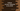# Python program to convert character to its ASCII value## Introduction :

In this tutorial, we will learn how to find the ASCII value of a character in python. The user will enter one character and our program will print the ASCII value.

ASCII or American Standard Code for Information Interchange is the standard way to represent each character and symbol with a numeric value. This example will show you how to print the ASCII value of a character.

## ord() function :

Python comes with one built-in method ord to find out the Unicode value of a character. The syntax of this method is as below :

``ord(c)``

This method takes one character as a parameter and returns the Unicode value of that character.

### Python program to convert character to its ASCII :

``````c = input("Enter a character : ")

print("The ASCII value of {} is {}".format(c,ord(c)))``````

As you can see that we are using ord method to find out the ASCII value of the user-provided character. The character is read using the input() _method and stored in the variable _‘c’.

### Sample Output :

``````Enter a character : a
The ASCII value of a is 97

Enter a character : A
The ASCII value of A is 65

Enter a character : #
The ASCII value of # is 35

Enter a character : *
The ASCII value of * is 42

Enter a character : \$
The ASCII value of \$ is 36

Enter a character : )
The ASCII value of ) is 41``````